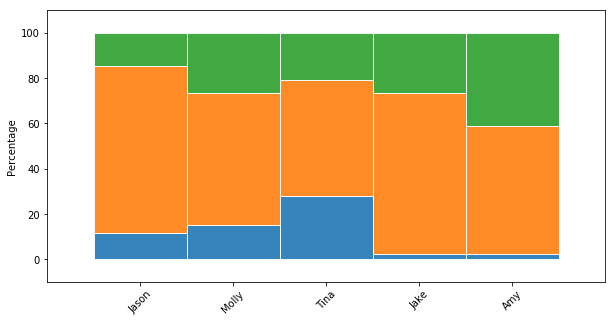How to generate stacked BAR plot in Python?
DATA VISUALIZATION DATA CLEANING PYTHON DATA MUNGING MACHINE LEARNING RECIPES PANDAS CHEATSHEET     ALL TAGS

# How to generate stacked BAR plot in Python?

This recipe helps you generate stacked BAR plot in Python

0
This python source code does the following : 1. Creates and converts data dictionary into dataframe 2. Plots stack bar graphs using matplotlib for visualization
In :
```## How to generate stacked BAR plot in Python
def Snippet_119():
print()
print(format('How to generate stacked BAR plot in Python','*^82'))

import warnings
warnings.filterwarnings("ignore")

import pandas as pd
import matplotlib.pyplot as plt

# Create dataframe
raw_data = {'first_name': ['Jason', 'Molly', 'Tina', 'Jake', 'Amy'],
'pre_score': [4, 24, 31, 2, 3],
'mid_score': [25, 94, 57, 62, 70],
'post_score': [5, 43, 23, 23, 51]}
df = pd.DataFrame(raw_data, columns = ['first_name', 'pre_score', 'mid_score', 'post_score'])
print(); print(df)

# Create a figure with a single subplot
f, ax = plt.subplots(1, figsize=(10,5))

# Set bar width at 1
bar_width = 1

# positions of the left bar-boundaries
bar_l = [i for i in range(len(df['pre_score']))]

# positions of the x-axis ticks (center of the bars as bar labels)
tick_pos = [i+(bar_width/2)-0.5 for i in bar_l]

# Create the total score for each participant
totals = [i+j+k for i,j,k in zip(df['pre_score'], df['mid_score'], df['post_score'])]

# Create the percentage of the total score the pre_score value for each participant was
pre_rel = [i / j * 100 for  i,j in zip(df['pre_score'], totals)]

# Create the percentage of the total score the mid_score value for each participant was
mid_rel = [i / j * 100 for  i,j in zip(df['mid_score'], totals)]

# Create the percentage of the total score the post_score value for each participant was
post_rel = [i / j * 100 for  i,j in zip(df['post_score'], totals)]

# Create a bar chart in position bar_1
ax.bar(bar_l, pre_rel, label='Pre Score', alpha=0.9, width=bar_width, edgecolor='white')

# Create a bar chart in position bar_1
ax.bar(bar_l, mid_rel, bottom=pre_rel, label='Mid Score', alpha=0.9, width=bar_width,
edgecolor='white')

# Create a bar chart in position bar_1
ax.bar(bar_l, post_rel, bottom=[i+j for i,j in zip(pre_rel, mid_rel)],
label='Post Score', alpha=0.9, width=bar_width, edgecolor='white')

# Set the ticks to be first names
print(tick_pos)
plt.xticks(tick_pos, df['first_name'])
ax.set_ylabel("Percentage")
ax.set_xlabel("")

# Let the borders of the graphic
plt.xlim([min(tick_pos)-bar_width, max(tick_pos)+bar_width])
plt.ylim(-10, 110)

# rotate axis labels
plt.setp(plt.gca().get_xticklabels(), rotation=45)
plt.show()

Snippet_119()
```
```********************How to generate stacked BAR plot in Python********************

first_name  pre_score  mid_score  post_score
0      Jason          4         25           5
1      Molly         24         94          43
2       Tina         31         57          23
3       Jake          2         62          23
4        Amy          3         70          51
[0.0, 1.0, 2.0, 3.0, 4.0]
```#### Relevant Projects

##### Predict Employee Computer Access Needs in Python
Data Science Project in Python- Given his or her job role, predict employee access needs using amazon employee database.

##### Human Activity Recognition Using Smartphones Data Set
In this deep learning project, you will build a classification system where to precisely identify human fitness activities.

##### Learn to prepare data for your next machine learning project
Text data requires special preparation before you can start using it for any machine learning project.In this ML project, you will learn about applying Machine Learning models to create classifiers and learn how to make sense of textual data.

##### Natural language processing Chatbot application using NLTK for text classification
In this NLP AI application, we build the core conversational engine for a chatbot. We use the popular NLTK text classification library to achieve this.

##### Time Series Forecasting with LSTM Neural Network Python
Deep Learning Project- Learn to apply deep learning paradigm to forecast univariate time series data.

##### Sequence Classification with LSTM RNN in Python with Keras
In this project, we are going to work on Sequence to Sequence Prediction using IMDB Movie Review Dataset​ using Keras in Python.

##### German Credit Dataset Analysis to Classify Loan Applications
In this data science project, you will work with German credit dataset using classification techniques like Decision Tree, Neural Networks etc to classify loan applications using R.

##### Resume parsing with Machine learning - NLP with Python OCR and Spacy
In this machine learning resume parser example we use the popular Spacy NLP python library for OCR and text classification.

##### Credit Card Fraud Detection as a Classification Problem
In this data science project, we will predict the credit card fraud in the transactional dataset using some of the predictive models.

##### Predict Census Income using Deep Learning Models
In this project, we are going to work on Deep Learning using H2O to predict Census income.